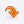UniversitiesLOGINCONTACTMost Recent ArticlesMost Rated ArticlesCategories

# Travel/ TourismGATE
GATE 2012 QUESTION TYPES
Author : Rajeswari
 Category : GATE posted Date :
 Total No.of views : Total No.of Comments : 0
Rating:3 / 5 (1 votes)

Tags :
Question types in GATE , Multiple choice Question in GATE, Common data questions

GATE 2012 QUESTION TYPES

Multiple Choice Questions

Multiple choice questions in all papers and sections will contain four answers, of which only one is correct. The types of questions in a paper may be based on following logic:

(i) Recall:

These are based on facts, principles, formulae or laws of the discipline. The candidate is expected to be able to obtain the answer either from his/her memory of the subject or at most from a one-line computation.

Example

Q. During machining maximum heat is produced

(A) in flank face       (B) in rake face

(C) in shear zone     (D) due to friction between chip and tool.

(ii) Comprehension:

These questions will test the candidate`s understanding of the basics of his/her field, by requiring him/her to draw simple conclusions from fundamental ideas.

Example

Q. A DC motor requires a starter in order

(A) to develop a starting torque

(B) to compensate for auxiliary field ampere turns

(C) to limit armature current at starting

(D) to provide regenerative braking

(iii) Application:

In these questions, the candidate is expected to apply his/her knowledge either through computation or by logical reasoning.

Example:

Q. The sequent depth ratio of a hydraulic jump in a rectangular channel is 16.48. The Froude number at the beginning of the jump is:

(A)  10.0  (B) 5.0

(C)  12.0  (D) 8.0

(iv) Analysis and Synthesis:
These can be linked questions, where the answer to the first question of the pair is required in order to answer its successor. Or these can be common data questions, in which two questions share the same data but can be solved independently of one another.

Common data questions

Multiple questions may be linked to a common data problem, passage and the like. Two or three questions can be formed from the given common data problem. Each question is independent and its solution obtainable from the above problem data/passage directly. (Answer of the previous question is not required to solve the next question). Each question under this group will carry two marks.

Example

Common Data, for instance, Questions 48 and 49 in main paper:

Let X and Y be jointly distributed random variables such that the conditional distribution of Y, given X=x, is uniform on the interval (x-1,x+1). Suppose E(X)=1 and Var(X)= 5/3

First question using common data:

Q.48 The mean of the random variable Y is

(A) 1/2  (B) 1   (C) 3/2       (D) 2

Second question using common data:

Q.49 The variance of the random variable Y is

(A) 1/2       (B) 2/3       (C) 1       (D) 2

Linked answer questions:

These questions are of problem solving type. A problem statement is followed by two questions based on the problem statement. The two questions are designed such that the solution to the second question depends upon the answer to the first one. In other words, the first answer is an intermediate step in working out the second answer. Each question in such linked answer questions will carry two marks.

Example:

Statement for Linked Answer Questions, for instance, for Questions 52 and 53 in Main Paper:

The open loop transfer function of a unity feedback control system is given by

First question of the pair:

Q.52 The value of K which will cause sustained oscillations in the closed loop system is

Second question of the pair:

Q.53 The frequency of sustained oscillations is

The questions based on the above four logics may be a mix of single stand alone statement / phrase / data type questions, combination of option codes type questions or match items types questions.

## CommentsReceive All Updates Via Facebook